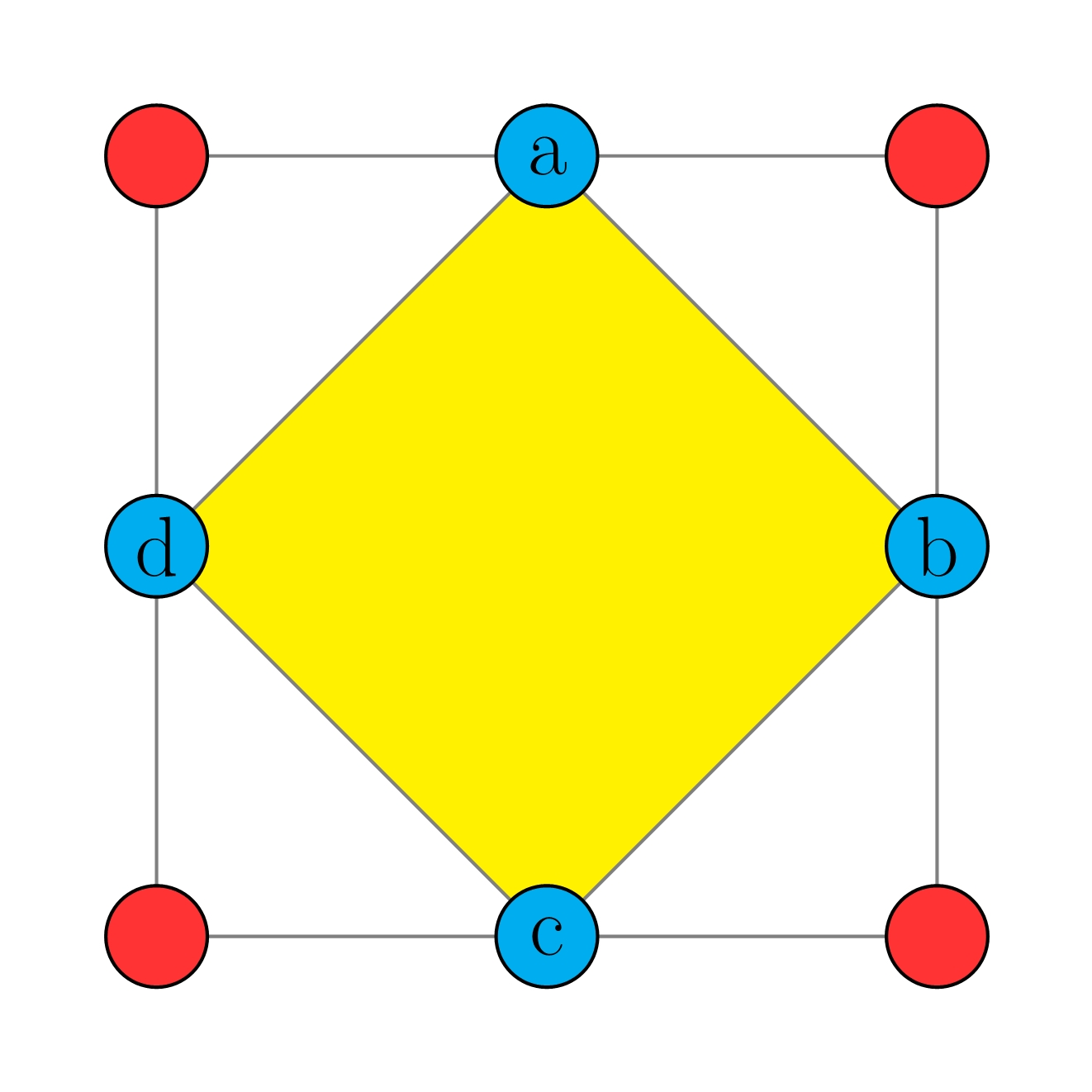# Question 1 of 303

Mark fills in each circle with a number from $1$, $2$, $3$, $\dots$, $8$, such that the sum of numbers at all corners of any triangle is 12. Find $(a+b+c+d)$.